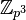# Classification of nilpotent Lie rings of prime-cube order

## Statement

Let$p$ be a prime number. There are, up to isomorphism, five possibilities for a nilpotent Lie ring of order$p^3$. Three of them are abelian and two are non-abelian.

For odd primes, we can use the Baer correspondence (a special case of the Lazard correspondence) to achieve a one-to-one correspondence between the nilpotent Lie rings of order$p^3$ and the groups of order$p^3$.

For the prime$p = 2$, although the number of nilpotent Lie rings equals the number of groups, we cannot use the Baer correspondence. More specifically, there is no natural way of matching the two non-abelian groups of order$p^3$ and the two non-abelian nilpotent Lie rings of order$p^3$.

### The three abelian Lie rings

The three abelian Lie rings are the Lie rings with trivial Lie bracket; these thus correspond to the abelian groups of order$p^3$. They are classified by the partitions of 3:

Partition of 3 Corresponding additive group of Lie ring
3 cyclic group of prime-cube order, denoted$C_{p^3}$ or$\mathbb{Z}_{p^3}$, or$\mathbb{Z}/p^3\mathbb{Z}$
2 + 1 direct product of cyclic group of prime-square order and cyclic group of prime order, denoted$C_{p^2} \times C_p$ or$\mathbb{Z}_{p^2} \times \mathbb{Z}_p$
1 + 1 + 1 elementary abelian group of prime-cube order, denoted$E_{p^3}$, or$C_p \times C_p \times C_p$, or$\mathbb{Z}_p \times \mathbb{Z}_p \times \mathbb{Z}_p$

### The two non-abelian nilpotent Lie rings

The two non-abelian nilpotent Lie rings are given as follows:

Lie ring name Presentation Nilpotency class Additive group Corresponding group under Baer correspondence in case of odd prime$p$
semidirect product of cyclic Lie ring of prime-square order and cyclic Lie ring of prime order$\langle a,b \mid p^2 a = pb = 0, [a,b] = a \rangle$ 2 direct product of cyclic group of prime-square order and cyclic group of prime order semidirect product of cyclic group of prime-square order and cyclic group of prime order
upper-triangular nilpotent matrix Lie ring:u(3,p)$\langle a,b,c \mid pa = pb = pc = 0, [a,c] = b, [a,b] = [b,c] = 0 \rangle$ 2 elementary abelian group of prime-cube order prime-cube order group:U(3,p); see Baer correspondence between u(3,p) and U(3,p)

## Proof

### First part of proof: crude description of center and quotient by center

Given: A prime number$p$, a nilpotent Lie ring$P$ of order$p^3$.

To prove: Either$P$ is abelian, or$Z(P)$ is cyclic of order$p$ and the quotient is an abelian Lie ring of order$p^2$ whose additive group is elementary abelian of order$p^2$.

Proof: Let$Z = Z(P)$ be the center of$P$.

Note: Some proof details need to be clarified, but the outline is complete and good enough for people thorough with group theory.

Step no. Assertion/construction Facts used Given data used Previous steps used Explanation
1$Z$ is nontrivial$P$ is nilpotent and nontrivial Given direct
2 The order of$Z$ cannot be$p^2$ PLACEHOLDER FOR INFORMATION TO BE FILLED IN: [SHOW MORE]$P$ has order$p^3$
3 The order of$Z$ is either$p$ or$p^3$ Steps (1), (2)
4 If$Z$ has order$p$, then$Z$ is cyclic of order$p$ and the quotient$P/Z$ is elementary abelian of order$p^2$.
5 If$Z$ has order$p^3$,$P$ is abelian
6 We get the desired result. Steps (4), (5) Step-combination.

### Third part of proof: classifying the non-abelian nilpotent Lie rings

Since we already know$Z(P)$ and$P/Z(P)$, we need to specify two things:

• The alternating bilinear map$P/Z(P) \times P/Z(P) \to Z(P)$ given by the Lie bracket: There is only one option for this (up to isomorphism) because$\bigwedge^2(P/Z(P))$ is isomorphic to$Z(P)$, and, up to conjugacy, there is a unique isomorphism.
• The nature of the additive group extension of$P/Z(P)$ on top of$Z(P)$: There are two possibilities for this: elementary abelian group of prime-square order (corresponding to the trivial or split extension)and direct product of cyclic group of prime-square order and cyclic group of prime order (corresponding to the nontrivial extensions).

Working out the corresponding Lie ring structures, we get upper-triangular nilpotent matrix Lie ring:u(3,p) (corresponding to the trivial extension) and semidirect product of cyclic Lie ring of prime-square order and cyclic Lie ring of prime order (corresponding to the nontrivial extension).